# 4.7. Consider a two-layer feedforward ANN with two inputs a and b, one hidden unit c, and one output unit d. This net...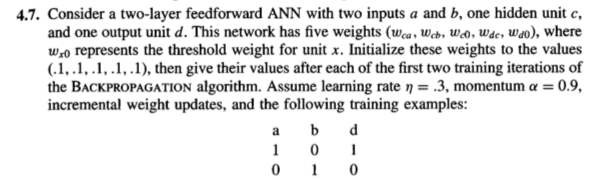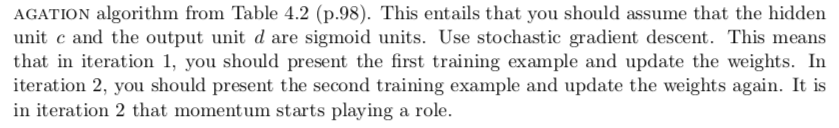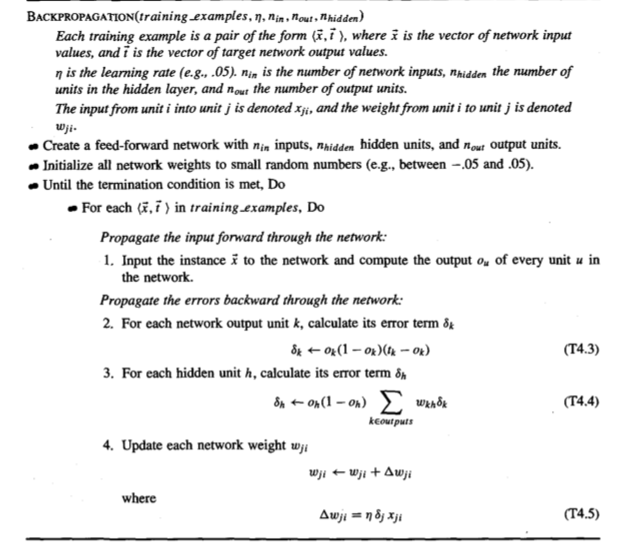4.7. Consider a two-layer feedforward ANN with two inputs a and b, one hidden unit c, and one output unit d. This network has five weights (wca, Wcb, Wco, Wse, Wao). where wro represents the threshold weight for unit x. Initialize these weights to the values (.1,.1,.1,.1,.1), then give their values after each of the first two training iterations of the BACKPROPAGATION algorithm. Assume learning rte '-.3, momentum α-: 0.9, incremental weight updates, and the following training examples: 0 1 0
AGATION algorithm from Table 4.2 (p.98). This entails that you should assume that the hiddern unit c and the output unit d are sigmoid units. Use stochastic gradient descent. This means that in iteration 1, you should present the first training example and update the weights. In iteration 2, you should present the second training example and update the weights again. It is in iteration 2 that momentum starts playing a role.
BACKPROPAGATION(training examples, η, nin , nout , n hidden) Each training example is a pair of the form (i,i ), where i is the vector of network input values, and i is the vector of target network output values. η is the learning rate (e.g., .05). nin is the number of network inputs, nhidden the number of units in the hidden layer, and nout the number of output units. The input from unit i into unit j is denoted xji, and the weight from unit i to unit j is denoted tD Create a feed-forward network with nin inputs, nhidden hidden units, and nour output units. Initialize all network weights to small random numbers (e.g., between -05 and.05). Until the termination condition is met, Do For each (z,i) in training examples, Do Propagate the input forward through the network 1. Input the instance i to the network and compute the output ou of every unit u in the network. errors backward through the network. 2. For each network output unit k, calculate its error term δk (T4.3) 3, For each hidden unit h , calculate its emor term (T4.4) keoutputs 4. Update each network weight wji where (T4.5)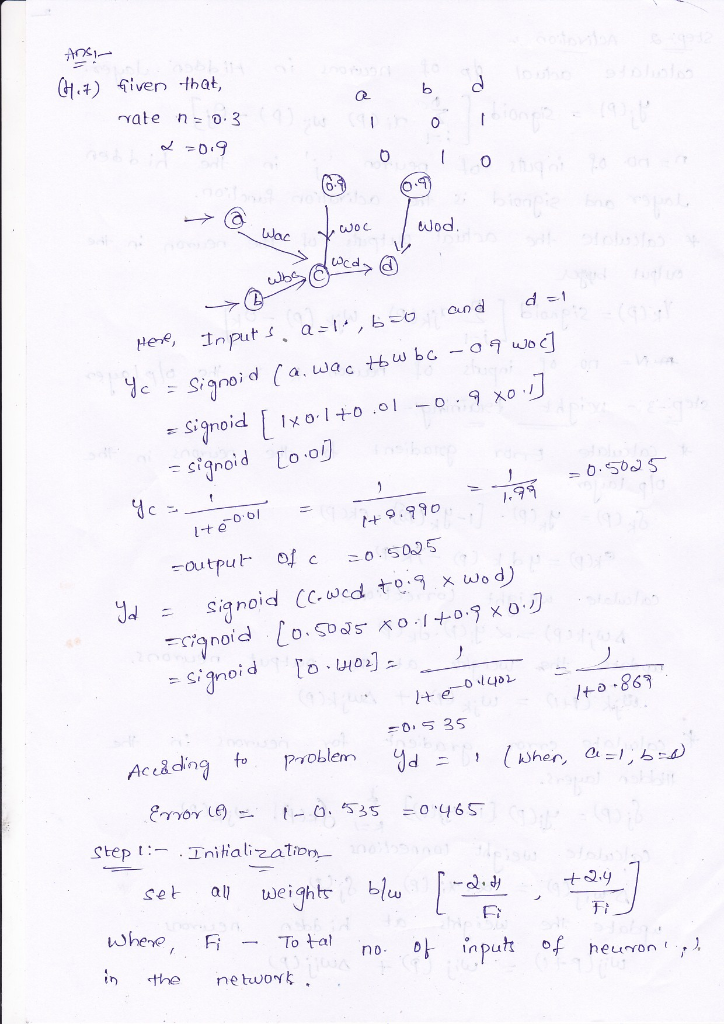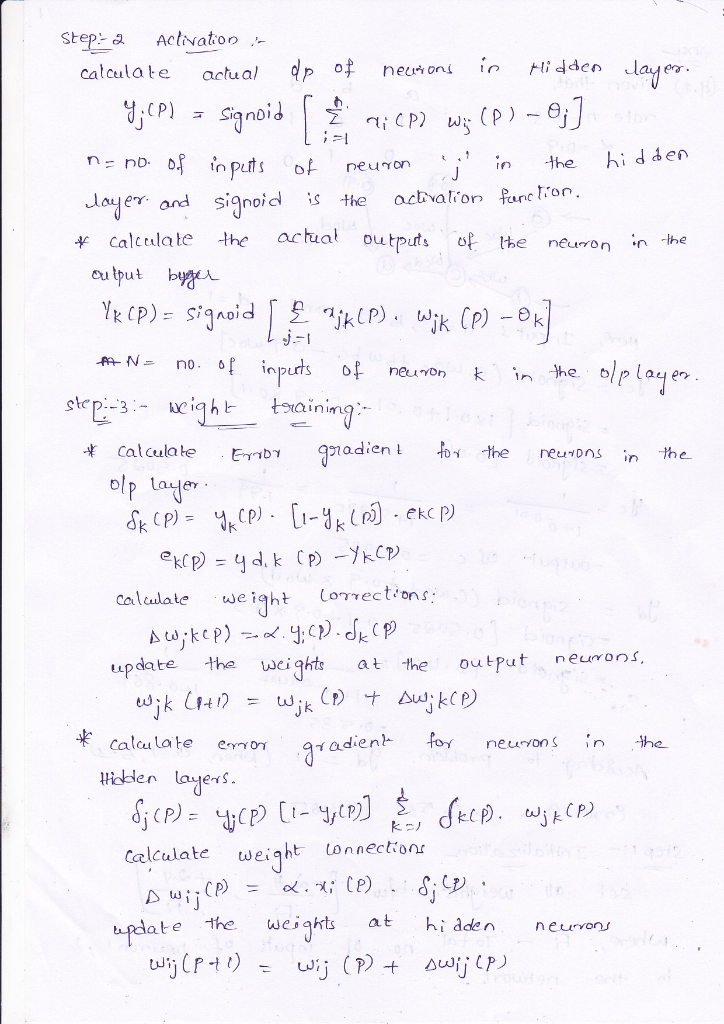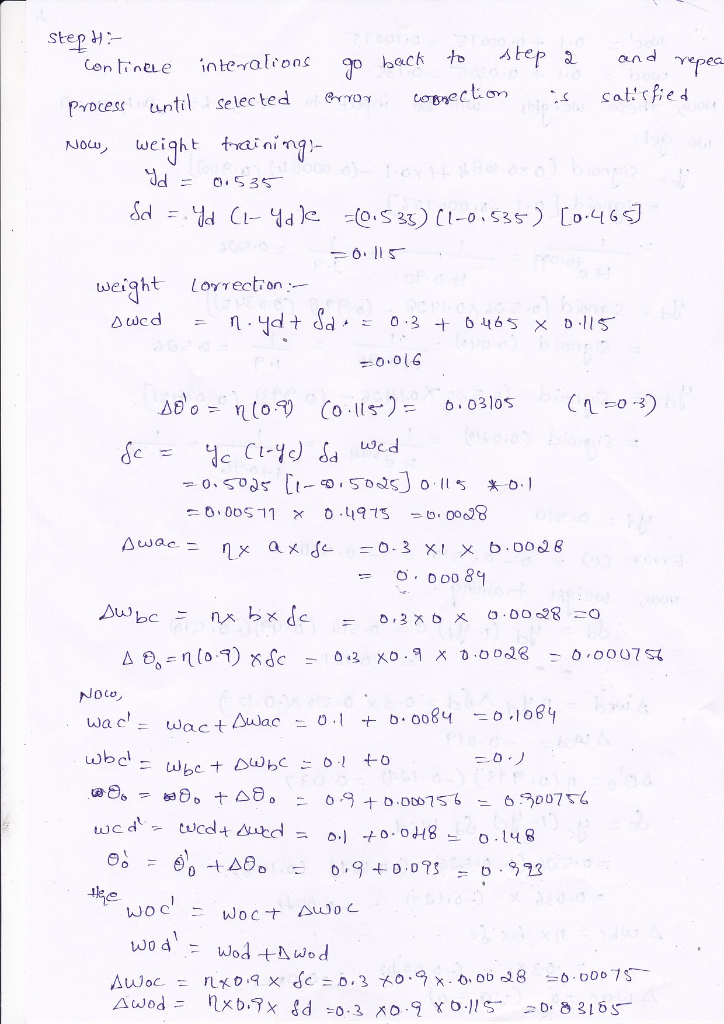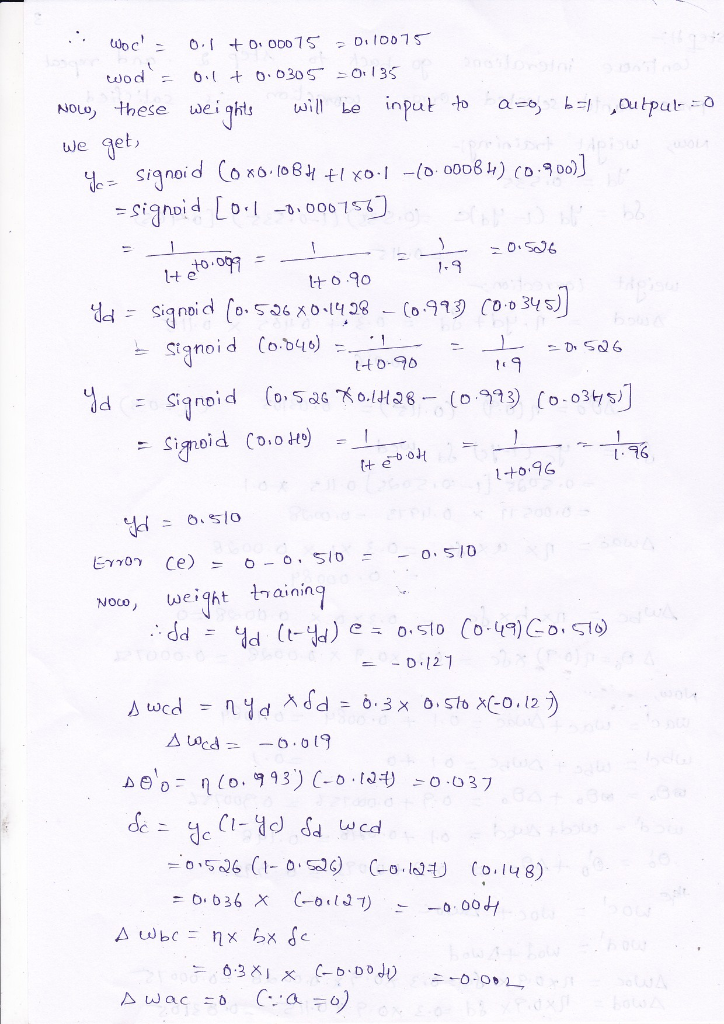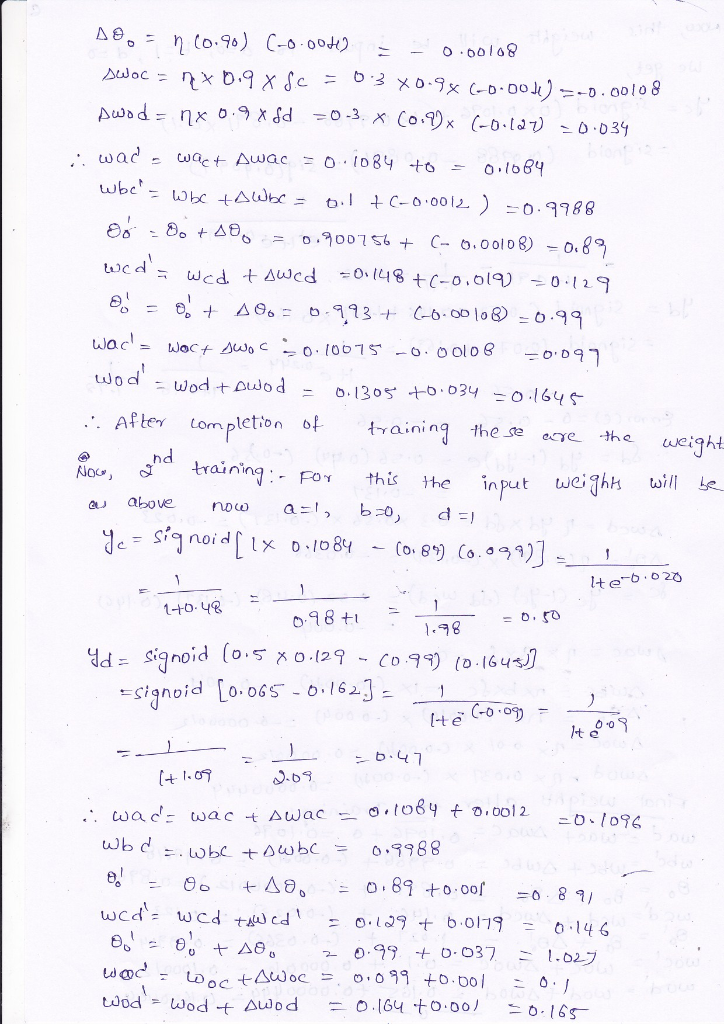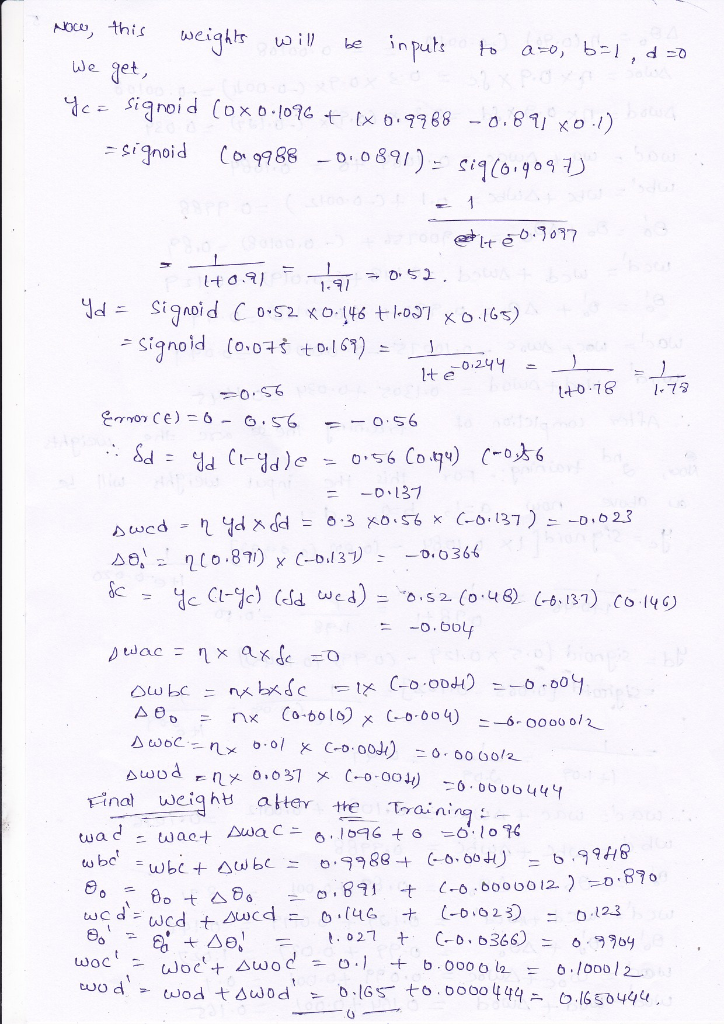##### Add Answer of: 4.7. Consider a two-layer feedforward ANN with two inputs a and b, one hidden unit c, and one output unit d. This net...
More Homework Help Questions Additional questions in this topic.